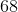# Common Core: 1st Grade Math : Understanding Place Value

## Example Questions

← Previous 1 3 4 5 6 7 8 9 23 24

### Example Question #1 : Understanding Place Value

What digit is in the tens place?NeitherExplanation:

The tens place is always the second number from the right.

### Example Question #4261 : Numbers And Operations

What digit is in the tens place?NeitherExplanation:

The tens place is always the second number from the right.

### Example Question #1 : Understand That A Two Digit Number Represents Tens And Ones: Ccss.Math.Content.1.Nbt.B.2:

What digit is in the tens place?NetiherExplanation:

The tens place is always the second number from the right.

### Example Question #4271 : Ssat Elementary Level Quantitative (Math)

What digit is in the tens place?NeitherExplanation:

The tens place is always the second number from the right.

### Example Question #442 : Whole And Part

What digit is in the tens place?NeitherExplanation:

The tens place is always the second number from the right.

### Example Question #451 : Whole And Part

What digit is in the tens place?NeitherExplanation:

The tens place is always the second number from the right.

### Example Question #452 : Whole And Part

What digit is in the ones place?NeitherExplanation:

The ones place is always the first number on the right.

### Example Question #453 : Whole And Part

What digit is in the ones place?NeitherExplanation:

The ones place is always the first number on the right.

### Example Question #451 : Whole And Part

What digit is in the ones place?NeitherExplanation:

The ones place is always the first number on the right.

### Example Question #454 : Whole And Part

What digit is in the ones place?Neither